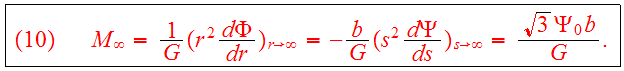# Lecture 6 Plummer's Spherical Model

## Plummer's Model

Consider a steady state star cluster with time-independentwhich implies a time-independent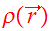. Introducing the notationand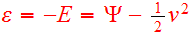, a very simple form for the distribution functionisEq.(1a,1b) defines Plummer's model. Calculate the density function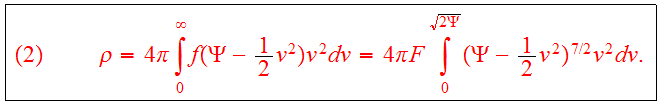If we make the substitution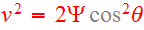,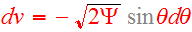, this becomeswhereIn Plummer's model the density rises as thepower of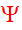when, and is, of course, zero whenWhen we use Eq.(3) to eliminate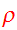from Poisson's equation, we find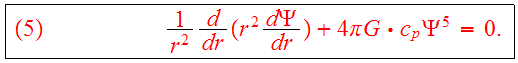If we eliminateandfrom Eq.(5) in favor of the rescaled variables,then Eq.(5) takes the simple form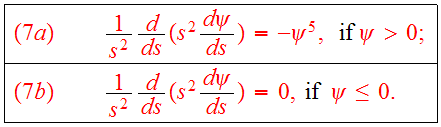The solution of Eqs.(7a,7b) is given byThe corresponding density isNote that the density is everywhere non-zero. The total mass is finite, however, with the value